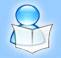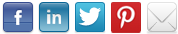A Collection of Informative and Interesting Articles
 HOME WANT AN ACCOUNT? SUBMIT ARTICLES TOP AUTHORS Debt Collections (Advt.)

# The Light Energy of Force

BY: Bob Ticer | Category: Others | Submitted: 2014-06-25 01:45:08Article Summary: "The concept of energy has a long historical development. Newton intuitively referred to it as force. How force is a form of energy is here explained. How it provides a causal explanation of gravity is also explained..."Total energy according to modern physics is conserved inasmuch as it neither increases nor decreases but only changes from one form to another. One particular form of energy according to Newtonian Mechanics is force, which Newton defined as a quantity of mass multiplied by the amount of its acceleration per time. Acceleration is a change in velocity. Velocity is defined as a change in speed or a change in direction or a combination of both change in speed and change in direction.

If quick enough, the amount of force changing the velocity of a small bug could be greater than that of a change in velocity of a locomotive.

How can energy be greater of a small bug than of a locomotive of far greater mass?

According to special relativity theory, it can only be greater if the relative speed of the bug is far greater than the relative speed of the locomotive, as total energy constitutes both the internal energy of mass and its kinetic energy of relative motion. Internal energy is the quantity of mass multiplied by light speed squared. Since the internal energy of either the bug or the locomotive is the same amount of light energy per mass, the internal energy of the locomotive of greater mass is also necessarily greater.

Could not a quicker change in velocity result in greater energy?

When the bug collides with the locomotive, the change in direction is not instantaneous; rather, there occurs an impulse for change that occurs in a manner consistent with conservation of energy. The degree of force is per time. The same change in velocity per less time equals more force, and an instantaneous change in velocity equates as an infinite force of energy, but to be consistent with conservation of energy the quicker time cannot be such that the force of the locomotive on the bug is greater than the total energy of the locomotive.

The significance of this result is with regard to how it pertains to other forms of energy in general. According to both Newtonian Mechanics and general relativity theory, for instance, inertial mass and gravitational mass are equivalent. Gravity as a form of energy is thus subject to the same conditions of energy as according to special relativity theory. Although general relativity merely explains gravity as according to space-time curvature due to the presence of mass, it does not explain what constitutes mass other than its modification according to relative space-time instead of according to Newton's concepts of absolute space and absolute time.

By the principle of equivalence, gravitational force compares with the kinetic energy of relative motion instead of the internal energy of the mass. However, gravity per mass in comparison to such other forces as electrostatics, magnetism and the kinetic energy of the locomotive is far weaker.

To explain this weaker gravitational force in view of conservation of energy, consider its impulse for changing momentum of other mass per time is weaker. In other words, it takes more time to occur. In effect, it is of longer range. However, it also propagates at light speed. How this is possible is according to how the gravitational radiation determines the change in space curvature through which it propagates.

According to general relativity, light speed is also slowed by the presence of a gravitational field. Space-time about the presence of mass thus has the same property of mass, that of inertia, which is a resistance to relative motion.

In my book, A Mystic History In Light Of Physics, a casual explanation of gravity is given as a vacuum effect due to an implosion of inertial space into mass emitting, in turn, gravitational radiation. In being consistent with conservation of energy, gravity interacts with inertial space as a long range change in its energy being absorbed by inertial space. The long range effects of absorbing gravitational radiation balance out in states of equilibrium. The decrease in the radiation propagated balances with the radiation created by mass. The range is consistent with the Hubble Constant whereby light from more distant stars is seen as red-shifted, as of less energy. The long range effect also constitutes a parameter that further determines an infinite universe of observational finite parts.

Rice is nice but Bob Ticer is nicer. Forty some years ago I had an idea of how to explain the cause of gravity. To complete the explanation, I felt it more necessary to examine the assumptions made by physicists rather than knowing the more complex mathematics that formulates the assumptions.

 Comment Comment By Comment Date

•   Stopping For the One

•   Is Every Friend Necessary ?

•   How to Be a Successful Clinical Psychologist

•   How to Keep Your Trees Happy

Latest Articles in "Others" category:
•   Understanding of the World

•   The Run, the Old Man and Me !

•   Ayahuasca ....here I Come !

•   A General Disussion

•   A Narrative on Animals and Birds

•   A Big Bang Debate

•   Another Day

Important Disclaimer: All articles on this website are for general information only and is not a professional or experts advice. We do not own any responsibility for correctness or authenticity of the information presented in this article, or any loss or injury resulting from it. We do not endorse these articles, we are neither affiliated with the authors of these articles nor responsible for their content. Please see our disclaimer section for complete terms.Copyright © 2010 saching.com - Do not copy articles from this website.
| Home | Disclaimer | Xhtml |